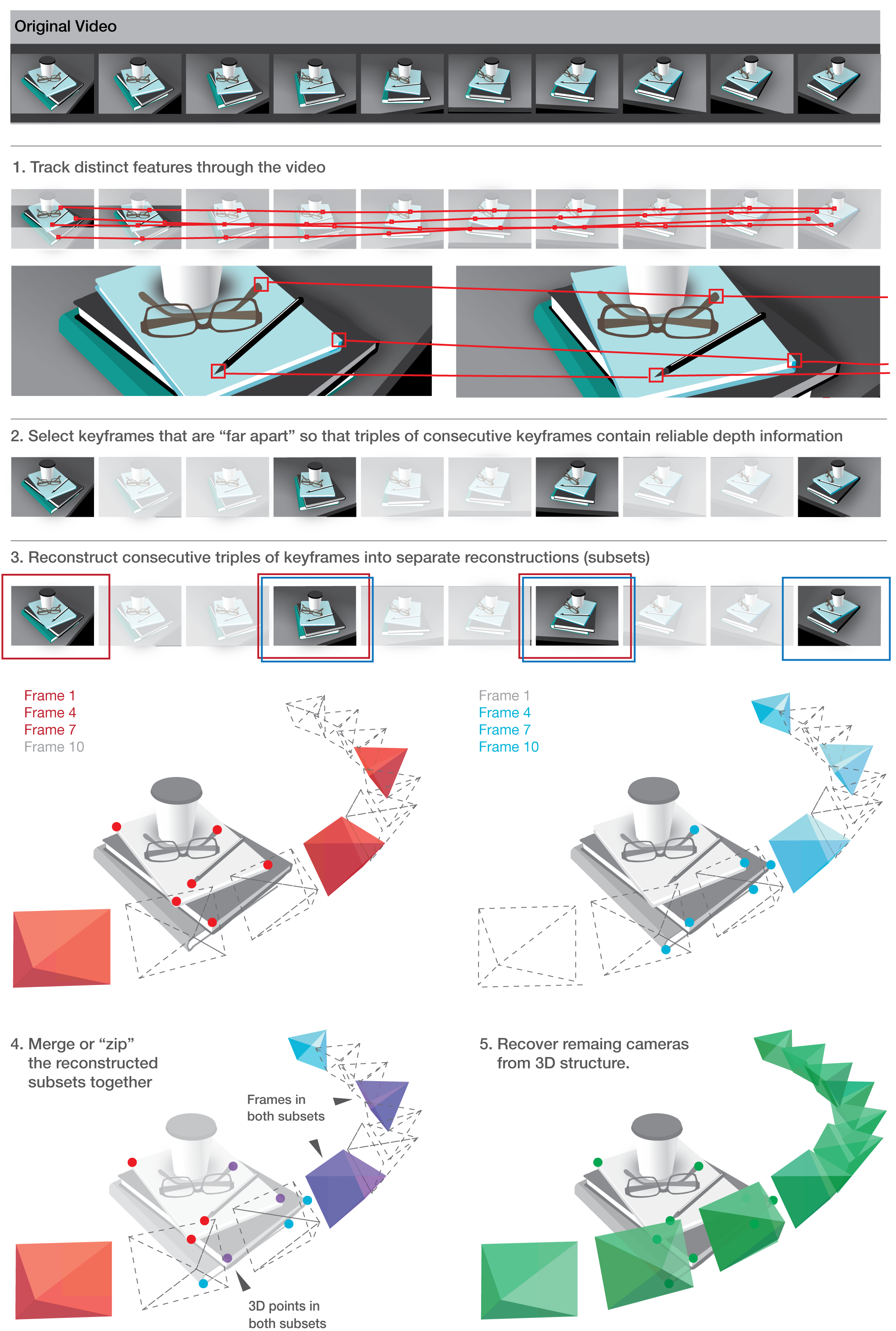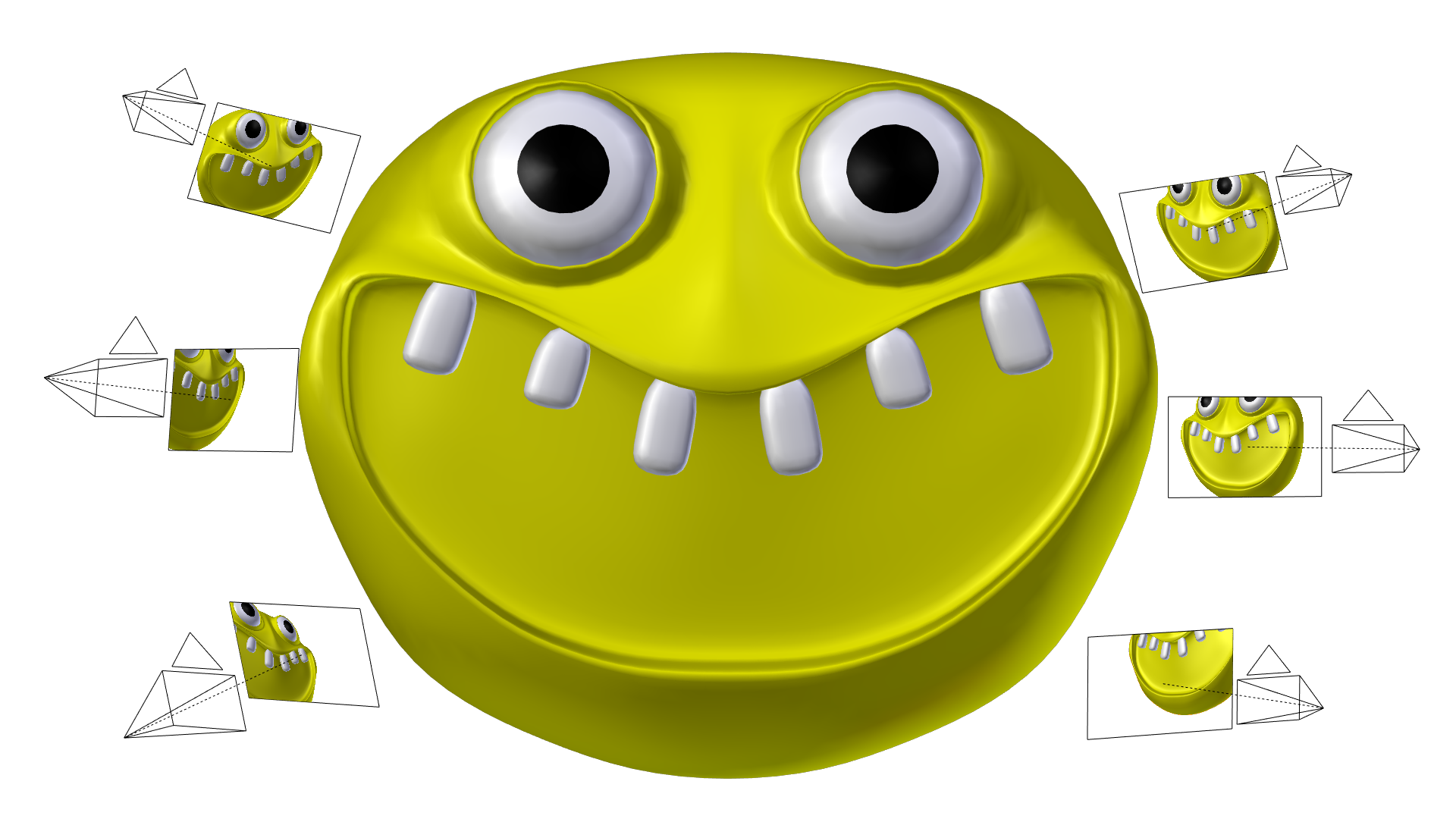# Master Thesis

## Agenda

• Last time (Recap)
• This time
• Paper details
• Conclusion / Feeling

# This time

### Ultra-Tightly Coupled Vision/GNSS for Automotive Applications[Aumayer, 2016]

Degree of Doctor of Philosophy (207 pages)

### Vision-Aided Pedestrian Navigation for Challenging GNSS Environments[Ruotsalainen, 2013]

Degree of Doctor of Science in Technology (181 pages)

### Integrating Vision Derived Bearing Measurements with Differential GPS for Vehicle-to-Vehicle Relative Navigation[Amirloo Abolfathi, 2015]

Degree of Master of Science (135 pages)

### sorry, too much :(

but: Some other time!

## Today

### Open Source 3D Reconstruction from Video[Mierle, 2008]

Master of Applied Science (124 pages)

## 1 Introduction

### 1 Introduction

Motivation:
Increasing number of pictures

Contribution:
Fully open source (!), Blender, Evaluation tool for research

Term "matchmoving":
Used in film industry

### 1 Introduction

Requirements:
Free, easy, documented, auto-testing, automated

Related work:
Commercial (Boujou, PFTrack, Syntheyes, ...)
and Open (VXL, OpenCV)

Evaluation:
real (range scanning vs. optical flow)
and synthetic (Maya vs. Blender) data sets

## 2 Reconstruction without the math

### 2 Reconstruction without the math

Problem:
video of a scene -> determine pose of camera and location of some points

Solution:
at highest level see next picture

### 2 Reconstruction without the math### 2 Reconstruction without the math

Find "good" features (2D)

Track features across frames (2D)

Discussion: Points vs. lines or curves or planes

No clear "best" strategy to recover
cameras and structure

### 2 Reconstruction without the math

Closed-form-solutions### 2 Reconstruction without the math

Closed-form-solutions

• (A) 7 * 2D-2D [=cams+points]
• (B) 6 * 2D-2D-2D [=cams+points]
• (C) 6 * 2D-2D-2D-2D [=cams+points]
• (D) 6 * 2D-3D (camera resectioning) [=1 cam]
• (E) 1 * 2D-2D (triangulation) [=1 point]

If perfect data: Use (B) then (D).

But...!

### 2 Reconstruction without the math

I) Tracks are unreliable: Outliers and drift

-> Solution: robust fitting (e.g. RANSAC)

-> Example: randomly pick six correspondences and try to solve for closed-form (compare 6 * 2D-2D-2D). Increase score of track if reprojection of 3D points into camera is close. Repeat to find best score.

### 2 Reconstruction without the math

II) Tracks are short: no longer than a few seconds

-> Solution: Merge multiple "triplets" of tracks into one

-> Pollefeys et al. (2004): Grow tracks by alternating closed-form-solutions

### 2 Reconstruction without the math

Critical issues:

• I) Insufficient camera motion between frames
-> Widen the baseline: Choose (key-)frames with strong depth
• II) Camera calibration (focal length, aspect ratio, and principal point)
-> Autocalibration always worse than manual calibration
• III) Barrels, pincushions, and Fisheyes
-> undistort lens or run bundle adjustment

## 3 The reconstruction system

### 3 The reconstruction system

For end user: Set of command line tools (9 to 10 steps)

$mplayer -vo pnm:pgm video.avi $ track sequence --pattern="%08d.pgm" --output="video.ts.json" --num features=500 --start=1 --end=100

$correct radial distortion --track="video.ts.json" --output="video corrected.ts.json" --intrinsics="CanonSD500.k.json" $ pick keyframes --track="video corrected.json" --output="video.kf.json"

$reconstruct subsets --track="video corrected.json" --bundle subsets=true --keyframes file="video.kf.json" --output="video.prs.json" --ransac rounds=100 $ merge subsets --track="video corrected.json" --subsets="video.prs.json" --output="video.pr.json" --bundle subsets=true

$resection --track="video corrected.json" --reconstruction="video.prs.json" --output="video resectioned.pr.json" --bundle subsets=true $ metric upgrade --track="video corrected.json" --reconstruction="video resectioned.prs.json" --intrinsics="CanonSD500.k.json" --output="video.mr.json"

$export blender --track="video corrected.json" --metric reconstruction="video.mr.json" --intrinsics="CanonSD500.k.json" --output="video.py" ### 3 The reconstruction system ## Preliminaries Projective Geometry It's really just homogeneous coordinates Points at infinity (line intersection) Represent all points from${\rm I\!R}^3$as projection in${\rm I\!R}^2$(up to a scale factor) =>$[4x; 4y; 4]^T$is the same as$[x; y; 1]^T$, (0 is infinity) ### 3 The reconstruction system ## Camera Projection Model Standard Euclidean projection$\begin{align} \begin{bmatrix} x \\ y \\ z \end{bmatrix} \end{align}$=$K[R(\hat{X}-C)]$,$\begin{align} \begin{bmatrix} \hat{x} \\ \hat{y} \end{bmatrix} \end{align}$=$\begin{align} \begin{bmatrix} x / z \\ y / z \end{bmatrix} \end{align}$translate, rotate 3D Points$\hat{X}$scale by intrinsics Matrix K ### 3 The reconstruction system ## Camera intrinsics$\begin{align} K &= \begin{bmatrix} focal_x && skew && center_x\\ 0 && focal_y && center_y \\ 0 && 0 && 1 \end{bmatrix} \end{align}$Without zooming, K is fixed for all images. ### 3 The reconstruction system ## Lens distortion$x^{''} = x^{'}(1 + k_1 r^2 + k_2 r^4) + 2p_1x^{'}y^{'} + p_2(r^2 + 2x^{'2})y^{''} = y^{'}(1 + k_1 r^2 + k_2 r^4) + 2p_2x^{'}y^{'} + p_1(r^2 + 2y^{'2})(r^2 = x^{'2} + y^{'2})$Normalized image coords:$x^{'}$,$y^{'}$radial distortion$k_{1,2}$tangential distortion$p_{1,2}$Perspective division -> distortion -> intrinsics ### 3 The reconstruction system ## Kanade-Lucas-Tomasi (KLT) tracker • 1.) Find outstanding features to track (extra talk!) • 2.) Track features across sequence KLT more efficient way to minimize:$\epsilon(\textbf{d}) = \frac{1}{2} \int\int_{Windows}^{} [J(\textbf{x} + \textbf{d}) - I(\textbf{x})]^2 weight(\textbf{x}) d\textbf{x} I(\cdot)$gray value frame1,$J(\cdot)$gray value frame2,$\textbf{x}$feature in frame1,$\textbf{d}$displacement Cross-Correlation-Search: Extremly slow! ### 3 The reconstruction system ## Kanade-Lucas-Tomasi (KLT) tracker uses Taylor expansion to reduce problem Task left: Solve a 2x2 linear system ### 3 The reconstruction system ## Radial unwarping When camera calibration information is available: • I) unwarp the video before tracking (using a Levenberg-Marquardt minimizer) • II) or: unwarp the correspondences after tracking If not: incorporate distortion terms into the final bundle adjustment (Calibration was not part of libMV, instead OpenCV was used) ### 3 The reconstruction system ## Keyframe selection Two metrics used by libMV: 1. count the number of tracks that survive from a reference frame 2. use a bucketing procedure in which the track count is constrained to image blocks ### 3 The reconstruction system ## Subset reconstruction three-frame six-point closed form solution described by Schaffalitzky et al. . fails miserably if one of the six points is in error ("outlier") ### 3 The reconstruction system therefore use closed form solution as a RANSAC driver 1. Randomly choose a subset of 6 points from correspondences ("inliers") 2. Fit this subset using the three-view six-point algorithm 3. Score the projective reconstruction and compare how well it matches rest of the data 4. Repeat 1.) and 2.) until probability of good set of points is found ### 3 The reconstruction system ## Calculating residual errors 1. Triangulate 2D point position from images 1 and 3 to get 3D point 2. Project 3D point into image 2 3. residual error is the difference between reprojected image location and tracked (measured) image location ### 3 The reconstruction system ## Scoring methods: many to choose from • RANSAC • LMedS • MSAC • AMLESAC • MLESAC (mixture model of 2D Gaussians) • AMLESAC2 (mixture of Rayleigh distributions, used in libMV) ### 3 The reconstruction system ## Residual error Assuming tracked points have isotropic additive Gaussian noise, Rayleigh distribution is a good model of the magnitude of reprojection errors.$p(x; \sigma_i, \sigma_o, \gamma) = \gamma p(x; \sigma_i) + (1 - \gamma) p (x; \sigma_o)x$=magnitude of residual error,$\sigma_i$variance of inliers,$\sigma_o$variance of outliers,$\gamma$inlier fraction AMLESAC2 fits above simultaneously via Expectation Maximization, scores on inlier variance ### 3 The reconstruction system ## Bundle adjustment after coarse reconstruction from closed-form solutions, adjust all the reconstruction parameters simultaneously to minimize reprojection error => nothing more than nonlinear minimization Many details which will be skipped here, can be a separate talk. Mostly continues with intelligent way to penalize errors This is an important step for reconstruction. ### 3 The reconstruction system ## Merging subsets libMV implements the one-frame overlap method (one camera in common) in Practice, merging projective reconstructions is quite unreliable. Hence, score subsets of 2 points from set of 3D correspondences and test repeatedly. ### 3 The reconstruction system ## Merging subsets Again, LMedS, RANSAC, MLESAC, etc. are used Nonlinear refinement is done using Levenberg-Marquardt Hierarchical merging (binary tree upwards) as opposed to sequential for errors not to accumulate at the end of the reconstruction ### 3 The reconstruction system ## Resectioning Recover remaining cameras to complete reconstruction Each correspondence produces two equations expressed as a matrix Compute missing camera matrix via SVD Use RANSAC again to remove outliers ### 3 The reconstruction system ## Metric upgrade "Projective reconstruction" is superset of "Euclidean (metric) reconstruction" Projective camera:$(K,R, \textbf(t))$Euclidean camera:$P = K[R|\textbf(t)]$(homogeneous matrix) ### 3 The reconstruction system ## Metric upgrade Goal: Find upgrading transformation ($H$) from projective reconstruction ($P$) and camera intrinsics ($K). Not supported back then: autocalibration (included now). ### 3 The reconstruction system ## Metric bundle adjustment Metric upgrade produces bad quality Reason: rotation components of camera matrices were forced to be orthogonal Effect: Drastically changes the projected image coordinates Solution: Bundle adjustment (same as projective BA, but using euler angles) ### 3 The reconstruction system ## Point cloud alignment for evaluation Evaluation with ground truth is hard, because of translation, scale, and rotation ambiguity (similarity transform) Example: Photo of car against white background -> is this a real car or a 10cm model? ### 3 The reconstruction system ## Point cloud alignment for evaluation Solution: Scale and align with ground truth data 1. Subtract the mean from both point clouds 2. Divide each point cloud by mean distance from origin 3. Orthogonal procrustes (use SVD):\min\limits_{R} = || A_{3xN} - RB_{3xN} ||^2\$
4. Combine into a single 4x4 transformation matrix

### 4 Evaluation of Multiview Algorithms

Synthetic: Point cloud from 3D scene, projected into image space, perturbed

Autocalibration: Final reconstruction more important to evaluate than recovered intrinsics (in practice)

Rendered images: Advantages like ground truth and control parameters (exposure, noise, distortion)

Blender community helped to provide renderings for evaluation

### 5 ResultsN-view instead of 3-view reconstruction gives better results

Manual selection of keyframes gives better results than automatic selection

### Conclusion

• a long read (124 pages)
• but a good one!

Pressure is growing!

### Feeling